# PSAT Math : How to multiply exponents

## Example Questions

← Previous 1 3

### Example Question #1 : Exponents

If (300)(400) = 12 * 10n, n =

4

12

2

7

3

4

Explanation:

(300)(400) = 120,000 or 12 * 104.

### Example Question #1 : Exponents

(2x103) x (2x106) x (2x1012) = ?

6x1021

6x1023

8x1021

8x1023

8x1021

Explanation:

The three two multiply to become 8 and the powers of ten can be added to become 1021.

### Example Question #1 : How To Multiply Exponents

Which of the following is equivalent to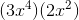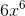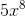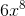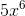Explanation: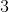and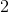can be multiplied together to give you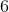which is the first part of our answer. When you multiply exponents with the same base (in this case,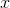), you add the exponents. In this case,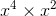should give us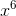because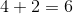. The answer is### Example Question #1 : How To Multiply Exponents

If 3x = 27, then 22x = ?

8

64

3

32

9

64

Explanation:
1. Solve for x in 3x = 27. x = 3 because 3 * 3 * 3 = 27.
2. Since x = 3, one can substitute x for 3 in 22x
3. Now, the expression is 22*3
4. This expression can be interpreted as 22 * 2* 22. Since 22 = 4, the expression can be simplified to become 4 * 4 * 4 = 64.
5. You can also multiply the powers to simplify the expression. When you multiply the powers, you get 26, or 2 * 2 * 2 * 2 * 2 * 2
6. 2= 64.

### Example Question #1 : Exponents

Find the value of x such that:

8x-3 = 164-x

7/2

4

25/7

19/4

11/3

25/7

Explanation:

In order to solve this equation, we first need to find a common base for the exponents. We know that 23 = 8 and 24 = 16, so it makes sense to use 2 as a common base, and then rewrite each side of the equation as a power of 2.

8x-3 = (23)x-3

We need to remember our property of exponents which says that (ab)c = abc.

Thus (23)x-3 = 23(x-3) = 23x - 9.

We can do the same thing with 164-x.

164-x = (24)4-x = 24(4-x) = 216-4x.

So now our equation becomes

23x - 9 = 216-4x

In order to solve this equation, the exponents have to be equal.

3x - 9 = 16 - 4x

7x - 9 = 16

7x = 25

Divide by 7.

x = 25/7.

### Example Question #1 : Exponents

Which of the following is equal to 410 + 410 + 410 + 410 + 411?

240

223

260

215

250

223

Explanation:

We can start by rewriting 411 as 4 * 410. This will allow us to collect the like terms 410 into a single term.

410 + 410 + 410 + 410 + 411

= 410 + 410 + 410 + 410 + 4 * 410

= 8 * 410

Because the answer choices are written with a base of 2, we need to rewrite 8 and 4 using bases of two. Remember that 8 = 23, and 4 = 22.

8 * 410

= (23)(22)10

We also need to use the property of exponents that (ab)c = abc. We can rewrite (22)10 as 22x10 = 220.

(23)(22)10

= (23)(220)

Finally, we must use the property of exponents that a* ac = ab+c.

(23)(220) = 223

### Example Question #481 : Algebra

If 3 + 3n+3 = 81, what is 3n+2 ?

18

26

3

81

9

26

Explanation:

3 + 3n+3 = 81

In this equation, there is a common factor of 3, which can be factored out.

Thus, 3(1 + 3n+2) = 81

Note: when 3 is factored out of 3n+3, the result is 3n+2 because (3n+3 = 31 * 3n+2). Remember that exponents are added when common bases are multiplied.  Also remember that 3 = 31.

3(1 + 3n+2) = 81

(1 + 3n+2) = 27

3n+2 = 26

Note: do not solve for n individually.  But rather seek to solve what the problem asks for, namely 3n+2.

### Example Question #2 : Exponents

If f(x) = (2 – x)(x/3), and 4n = f(10), then what is the value of n?

–2

0

5

–5

2

5

Explanation:

First, let us use the definiton of f(x) to find f(10).

f(x) = (2 – x)(x/3)

f(10) = (2 – 10)(10/3)

= (–8)(10/3)

In order to evaluate the above expression, we can make use of the property of exponents that states that abc = (ab)c = (ac)b.

(–8)(10/3) = (–8)10(1/3) = ((–8)(1/3))10.

(–8)(1/3) requires us to take the cube root of –8. The cube root of –8 is –2, because (–2)3 = –8.

Let's go back to simplifying ((–8)(1/3))10.

((–8)(1/3))10 = (–2)10 = f(10)

We are asked to find n such that 4n = (–2)10. Let's rewrite 4n with a base of –2, because (–2)2 = 4.

4n = ((–2)2)n = (–2)2n = (–2)10

In order to (–2)2n = (–2)10, 2n must equal 10.

2n = 10

Divide both sides by 2.

n = 5.

### Example Question #2 : Exponents

What is the value of n that satisfies the following equation?

2n·4n·8n·16 = 2-n·4-n·8-n

1/3

–2/3

–1/3

2/3

0

–1/3

Explanation:

In order to solve this equation, we are going to need to use a common base. Because 2, 4, 8, and 16 are all powers of 2, we can rewrite both sides of the equation using 2 as a base. Since 22 = 4, 23 = 8, and 24 = 16, we can rewrite the original equation as follows:

2n4n8n16 = 2n 4n 8n

2n(22)n(23)n(24) = 2n(22)n(23)n

Now, we will make use of the property of exponents which states that (ab)c = abc.

2n(22n)(23n)(24) = 2n(22n)(23n)

Everything is now written as a power of 2. We can next apply the property of exponents which states that abac = ab+c.

2(n+2n+3n+4) = 2(–n + –2n + –3n)

We can now set the exponents equal and solve for n.

+ 2+ 3+ 4 = –n + –2n + –3n

Let's combine the n's on both sides.

6n + 4 = –6n

12n + 4 = 0

Subtract 4 from both sides.

12n = –4

Divide both sides by 12.

n = –4/12 = –1/3

### Example Question #2 : Exponents

If 1252x–4 = 6257–x, then what is the largest prime factor of x?

7

5

2

3

11

2

Explanation:

First, we need to solve 1252x–4 = 6257–x . When solving equations with exponents, we usually want to get a common base. Notice that 125 and 625 both end in five. This means they are divisible by 5, and they could be both be powers of 5. Let's check by writing the first few powers of 5.

51 = 5

52 = 25

53 = 125

54 = 625

We can now see that 125 and 625 are both powers of 5, so let's replace 125 with 53 and 625 with 54

(53)2x–4 = (54)7–x

Next, we need to apply the rule of exponents which states that (ab)c = abc .

53(2x–4) = 54(7–x)

We now have a common base expressed with one exponent on each side. We must set the exponents equal to one another to solve for x.

3(2x – 4) = 4(7 – x)

Distribute the 3 on the left and the 4 on the right.

6x – 12 = 28 – 4x

10x – 12 = 28

10x = 40

Divide by 10 on both sides.

x = 4

However, the question asks us for the largest prime factor of x. The only factors of 4 are 1, 2, and 4. And of these, the only prime factor is 2.